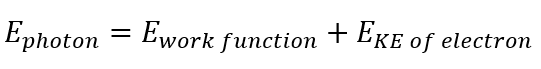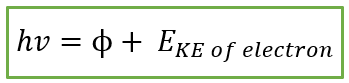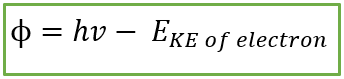# Problem: In order to comply with the requirement that energy be conserved, Einstein showed in the photoelectric effect that the energy of a photon (hν) absorbed by a metal is the sum of the work function (ϕ), which is the minimum energy needed to dislodge an electron from the metal’s surface, and the kinetic energy (Ek) of the electron: hν = ϕ + E k. When light of wavelength 358.1 nm falls on the surface of potassium metal, the speed (u) of the dislodged electron is 6.40 x 105 m/s. What is ϕ (in J) of potassium?

###### FREE Expert Solution

The mathematical representation of the photoelectric effect is:Since the energy of a photon (Ephoton) is also equal to (h∙v) where h is Planck’s constant (6.626x10-34 J∙s) and v is the frequency (in Hz or s-1), and work function can be denoted as ϕ, the photoelectric equation can be written as:Therefore, the equation for work function, ϕ is:###### Problem Details

In order to comply with the requirement that energy be conserved, Einstein showed in the photoelectric effect that the energy of a photon (hν) absorbed by a metal is the sum of the work function (ϕ), which is the minimum energy needed to dislodge an electron from the metal’s surface, and the kinetic energy (Ek) of the electron: hν = ϕ + E k. When light of wavelength 358.1 nm falls on the surface of potassium metal, the speed (u) of the dislodged electron is 6.40 x 105 m/s. What is ϕ (in J) of potassium?